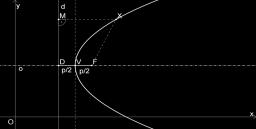# System: 4681

Solve the system:
(x + 5) (y-2) = (x-1) (y + 1)
(x + 1) (y + 1) = (x + 5) (y-1)

x = 2y-3

### Step-by-step explanation:Did you find an error or inaccuracy? Feel free to write us. Thank you!

Tips for related online calculators
Are you looking for help with calculating roots of a quadratic equation?
Do you have a linear equation or system of equations and looking for its solution? Or do you have a quadratic equation?